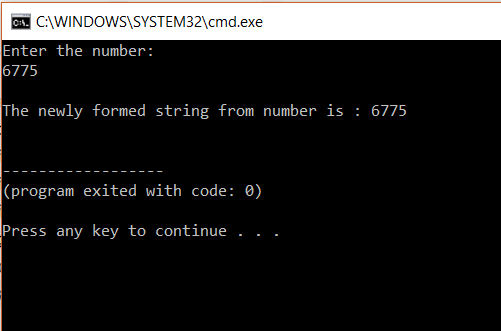# How To Convert Integer To String In C++?How To Convert Integer To String In C++?

Sometimes, while coding, we come across a problem where we require to convert a variable from integer to string. In this article we will learn how to covert integer to string in C++. Following pointers will be discussed in this article,

• Using String Streams
• Using To String Function
• Using Boost Lexical Cast

## Using String Streams

The first way is by using string streams, that is, the input or output string streams.

#### Convert Integer To String In C++: Sample Code

```#include<iostream>
#include <sstream>
#include <string>
using namespace std;
int main()
{
int num;
cout<<"Enter the number:&nbsp; "<<endl;
cin>>num;
ostringstream strg;
strg<< num;
string s1 = strg.str();
cout <<endl<< "The newly formed string from number is : ";
cout << s1 << endl;
return 0;
}
```

OutputExplanation

In the above program, we take an integer input from the user and convert it to string. We do this by using string streams method. We have two header files that are included, one is for string and the other is for string input/output streams. We have declared two variables. The first one is of type int. It is used to hold the number entered by the user.

We create a variable of output string stream class and create a variable str. We then pass the number stored in num to the output stream. Then we call the str() function to convert the number to string and print it.

Let us move on to the next topic of this article on ‘convert integer to string in C++’

## Using To String Function

The next method is using the string function to_string. Any data type can be converted to a string by using to_string.

#### Sample Code

```#include<iostream>
#include<string>
using namespace std;
int main()
{
int intVal;
cout << "Enter a Integer : "<<endl;
cin>>intVal;
string str = to_string(intVal);
cout << "The integer in string is : ";
cout << str << endl;
return 0;
}
```

OutputExplanation

In the above program, we take an integer input from the user and convert it to string. We do this by using to_string function. We have one header file that is included, it is for strings and the to_string function.

We accept a value from the user and store it in intVal. We then create a string variable str and assign the value of to_string() function, when intVal is passed.

We then print str and the integer is converted to a string.

Let us move on to the next topic of this article on ‘convert integer to string in C++’

## Using Boost Lexical Cast

Boost.LexicalCast can convert a number from strings to numeric and vice versa. It is present in “boost/lexical_cast.hpp” library. Here, we will convert a number to string using Boost.LexicalCast.

Sample Code

```#include<iostream>
#include <boost/lexical_cast.hpp>
#include <string>
using namespace std;
int main()
{
int m = 9487;
string str = boost::lexical_cast<string>(m);
cout << "The string value is : ";
cout << str << endl;
return 0;
}
```

Output

The string value is: 9487

Explanation

In the above program, we take an integer input from the user and convert it to string. We do this by using Boost.LexicalCast. We have two header file that is included, one is for strings and the other is for Boost.LexicalCast.

We assign m the value 9487. We create the string variable str and assign the value we get from Boost.LexicalCast to it. The conversion process takes place here. Then we print the output.

Thus we have come to an end of this article on ‘ ‘convert integer to string in C++’’. If you wish to learn more, check out the Java Training by Edureka, a trusted online learning company. Edureka’s Java J2EE and SOA training and certification course is designed to train you for both core and advanced Java concepts along with various Java frameworks like Hibernate & Spring.

Got a question for us? Please mention it in the comments section of this article  and we will get back to you as soon as possible.